# Selina Solutions Concise Mathematics Class 6 Chapter 15: Decimal Fractions Exercise 15(B)

Selina Solutions Concise Mathematics Class 6 Chapter 15 Decimal Fractions Exercise 15(B) help students, giving detailed steps to be followed in addition and subtraction of decimals. Subject experts at BYJU’S, help students with accurate answers for reference purposes while exam preparation. Selina Solutions is the best study material, which assists them to secure more marks in the exams. To boost the problem solving abilities of students, Selina Solutions Concise Mathematics Class 6 Chapter 15 Decimal Fractions Exercise 15(B), PDF links are mentioned here for free download.

## Selina Solutions Concise Mathematics Class 6 Chapter 15: Decimal Fractions Exercise 15(B) Download PDF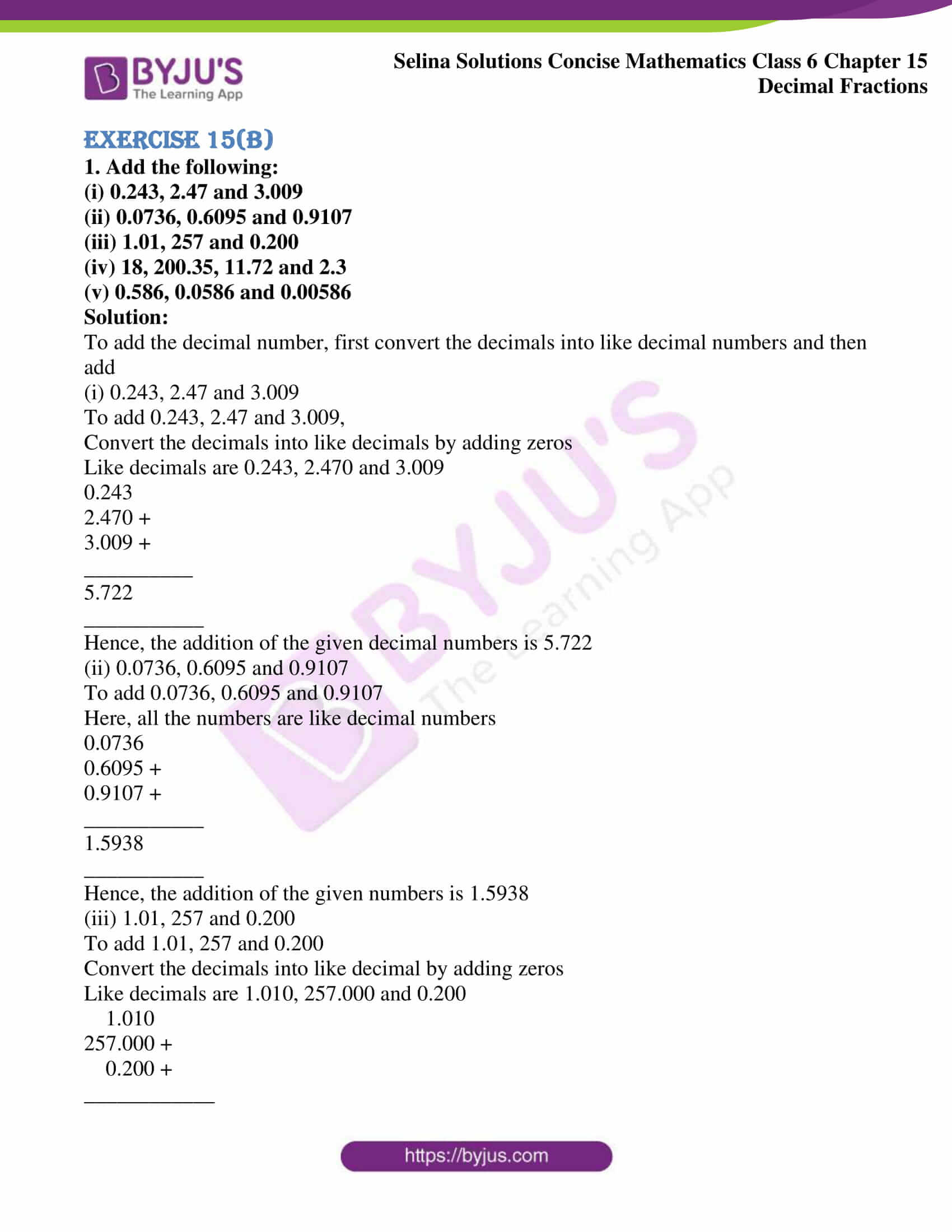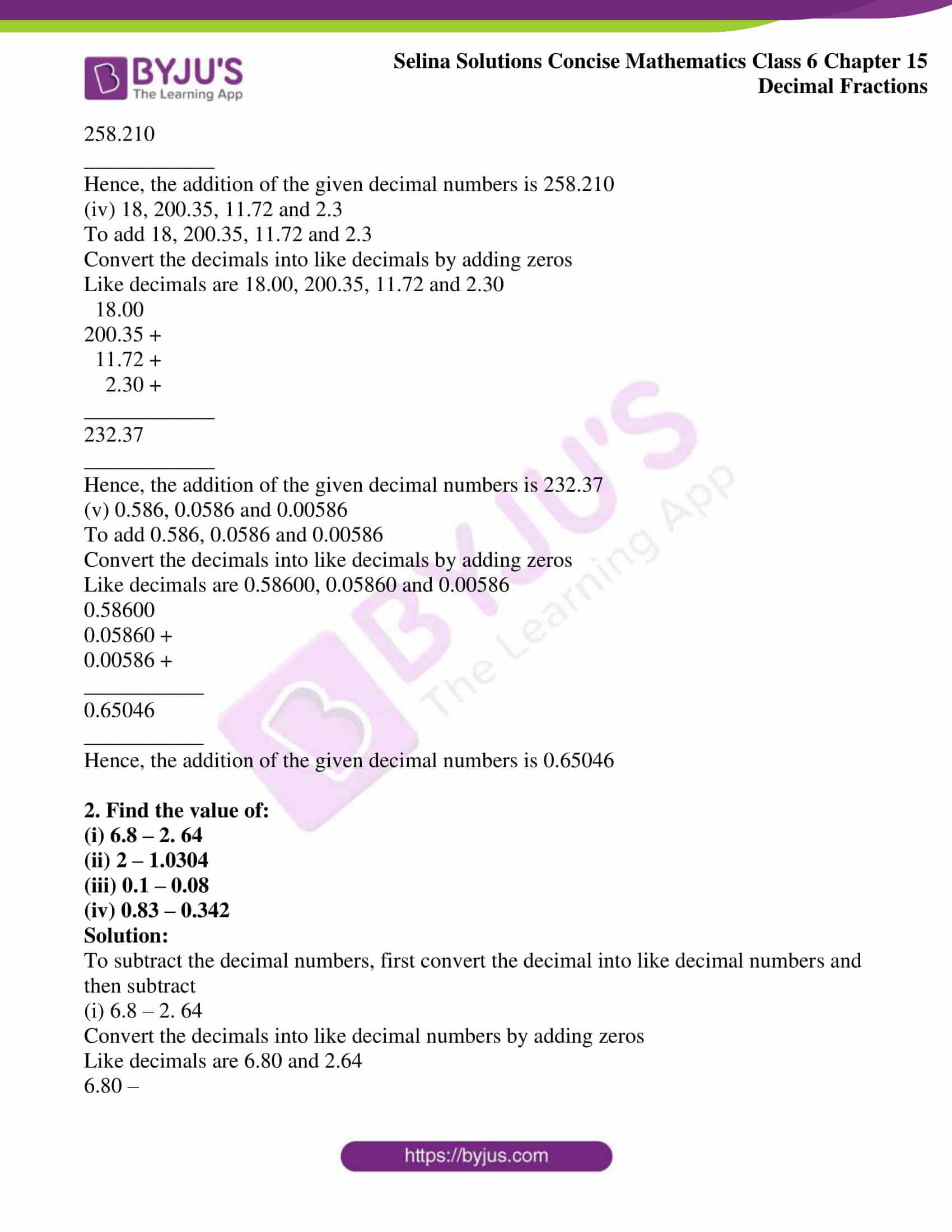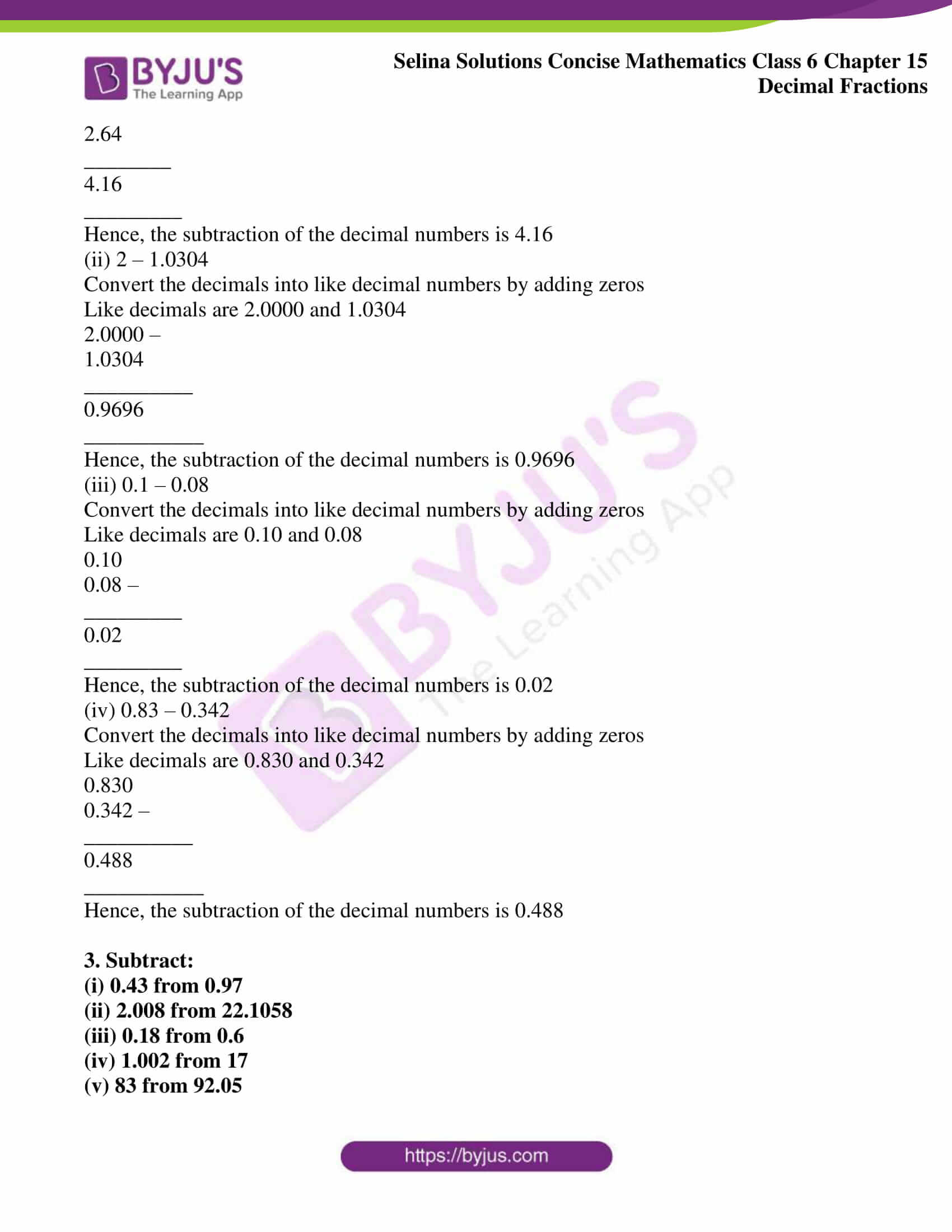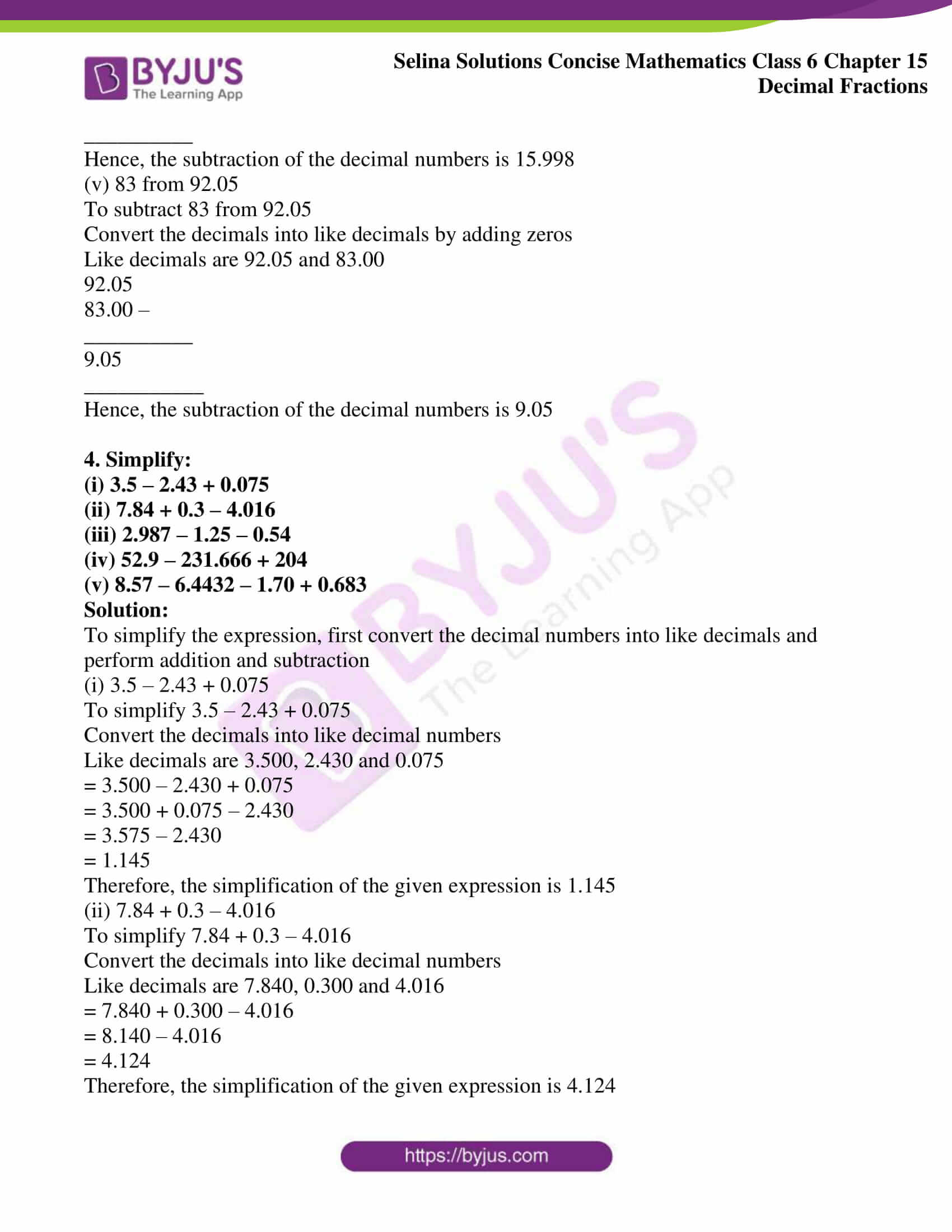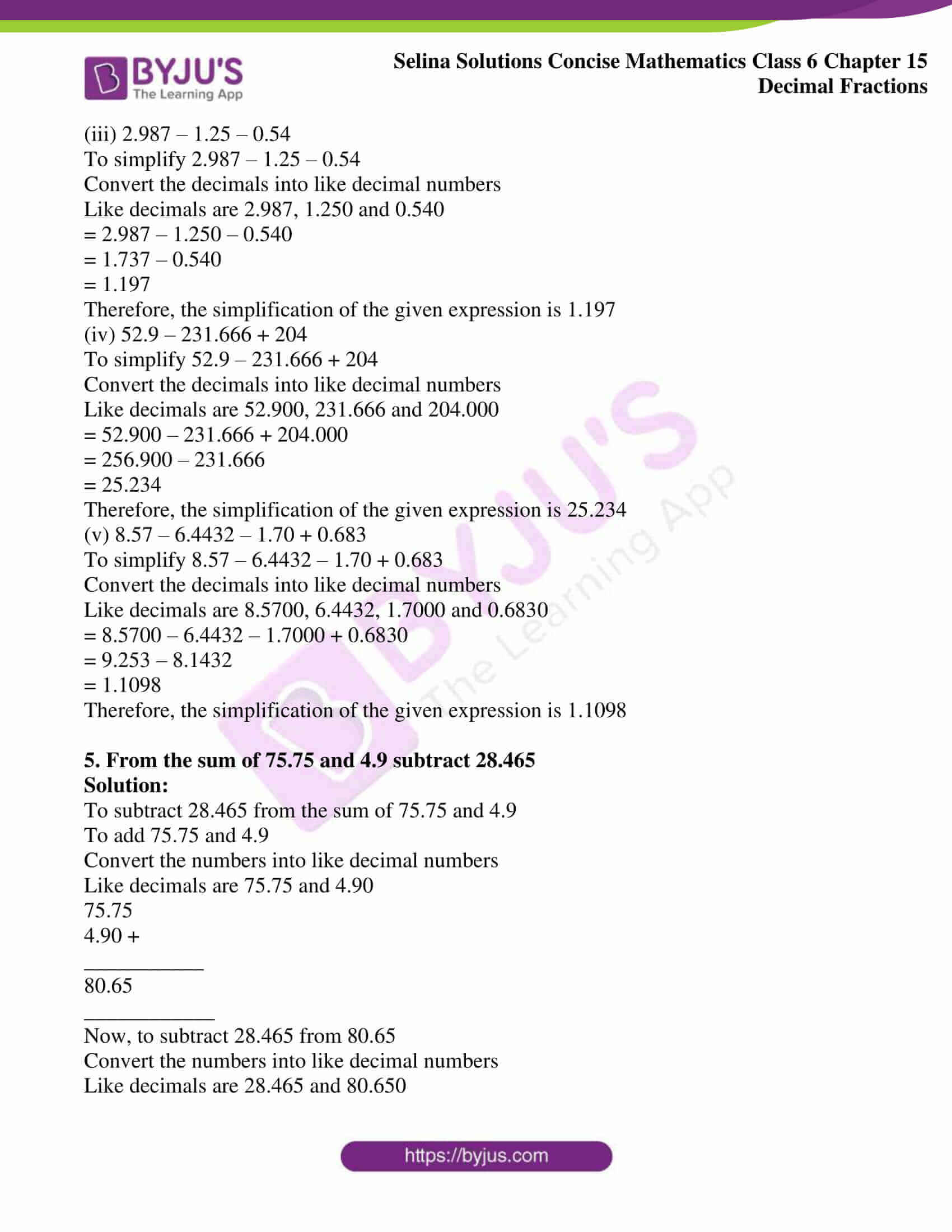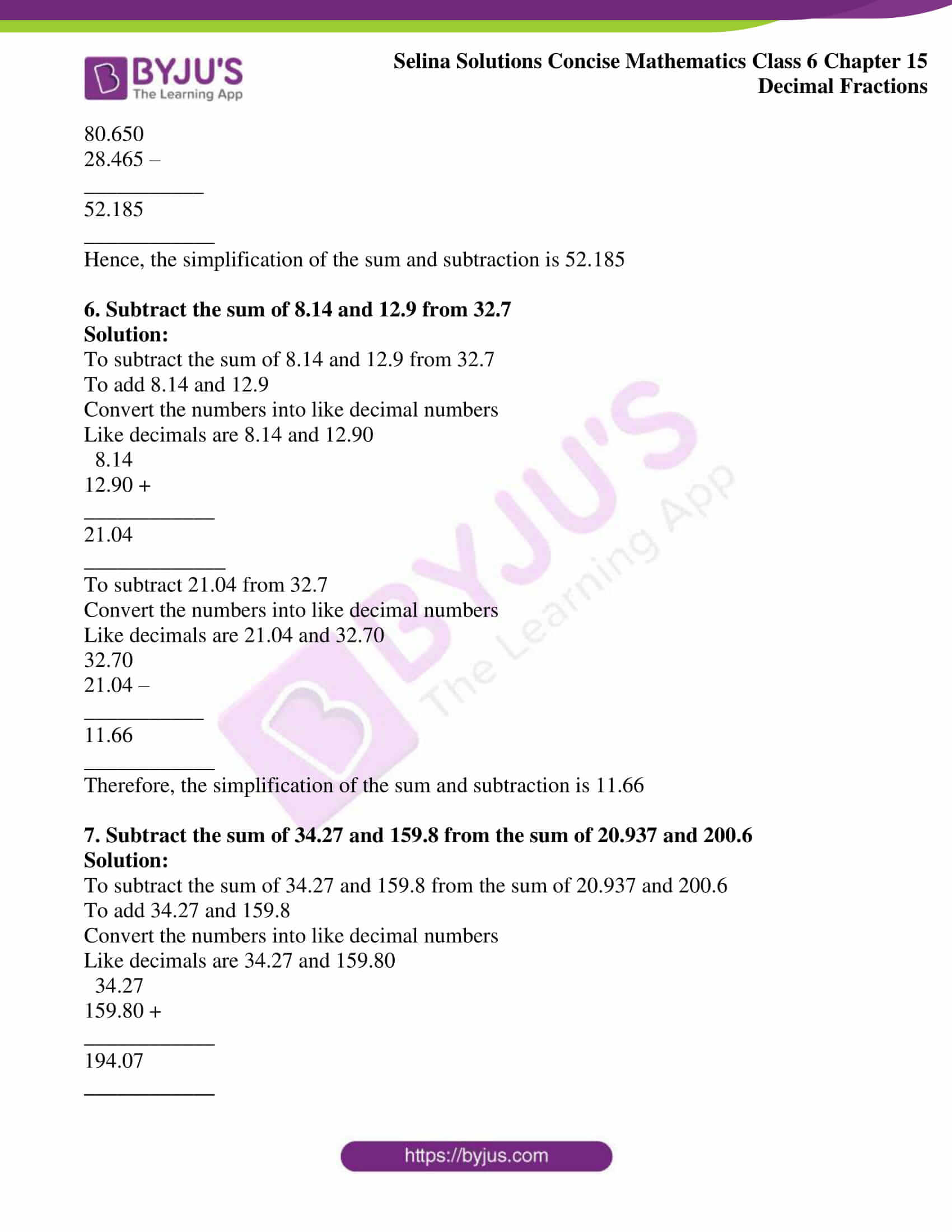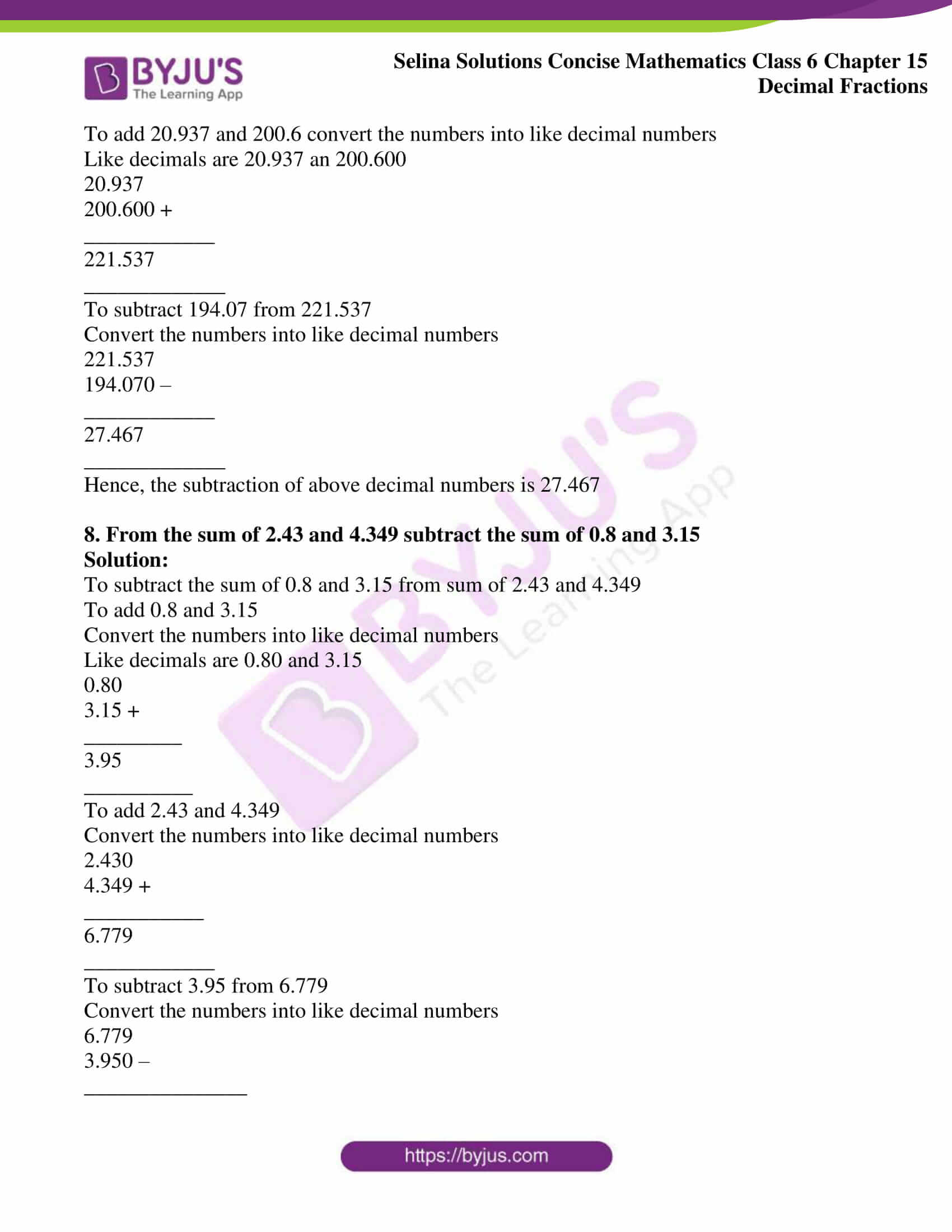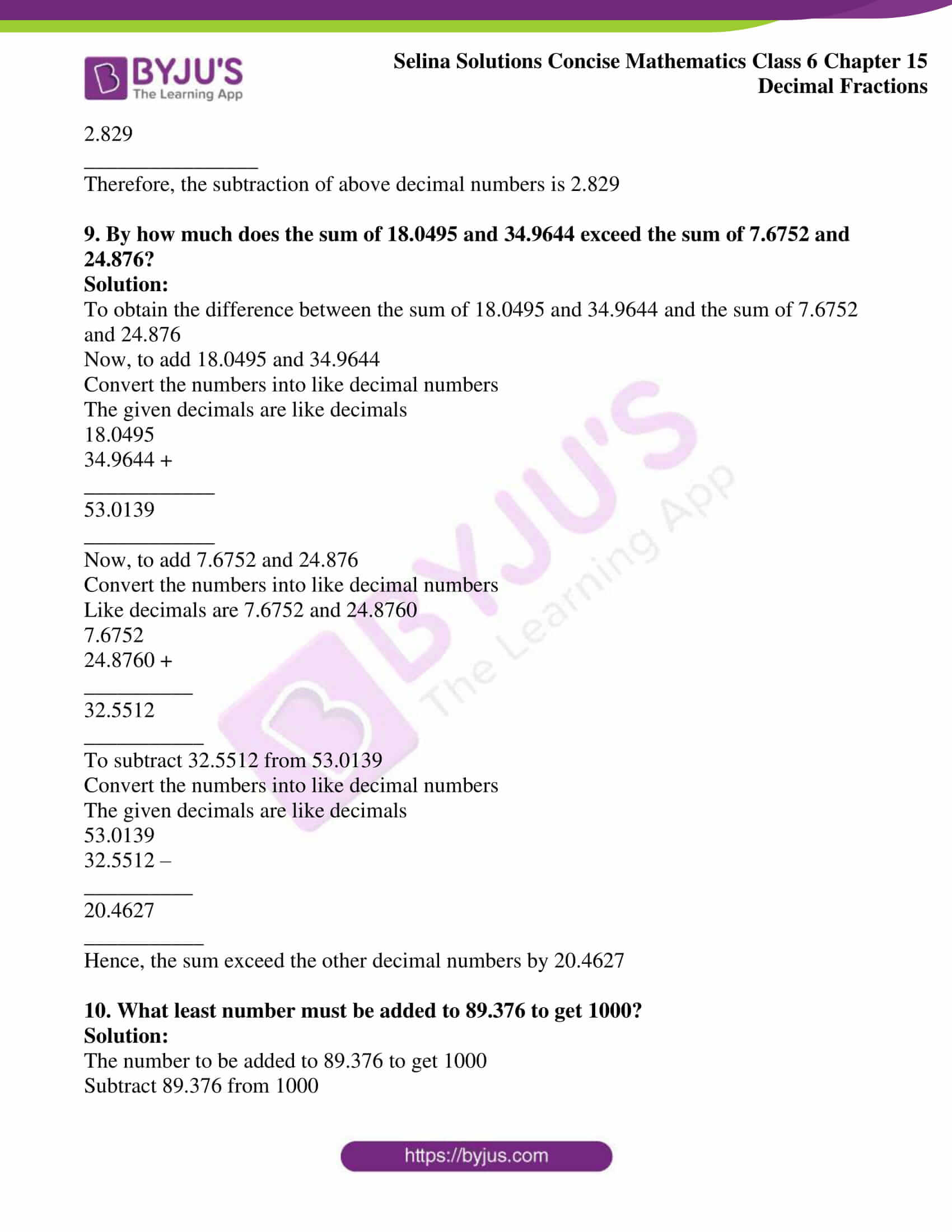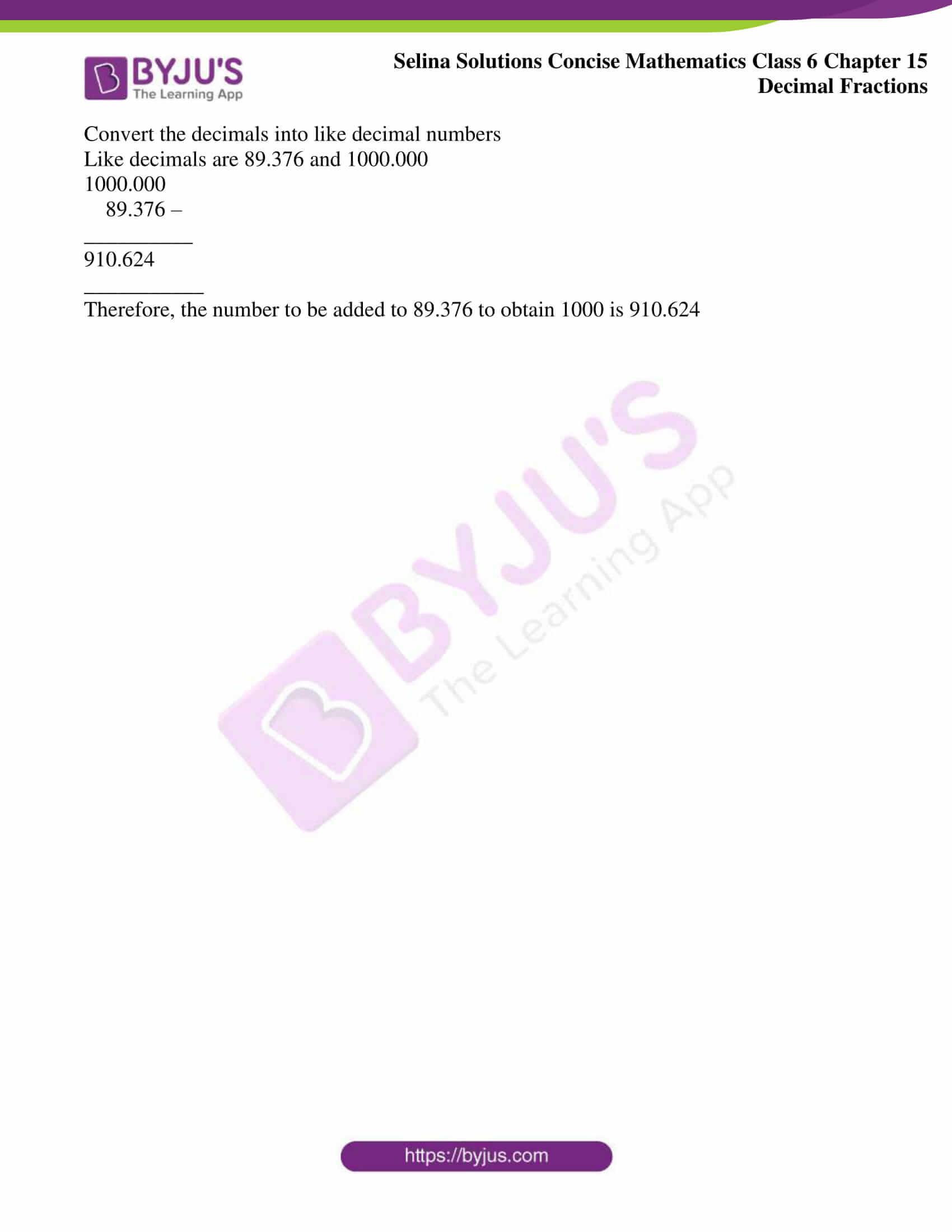### Access other exercises of Selina Solutions Concise Mathematics Class 6 Chapter 15: Decimal Fractions

Exercise 15(A) Solutions

Exercise 15(C) Solutions

Exercise 15(D) Solutions

Exercise 15(E) Solutions

### Access Selina Solutions Concise Mathematics Class 6 Chapter 15: Decimal Fractions Exercise 15(B)

Exercise 15(B)

(i) 0.243, 2.47 and 3.009

(ii) 0.0736, 0.6095 and 0.9107

(iii) 1.01, 257 and 0.200

(iv) 18, 200.35, 11.72 and 2.3

(v) 0.586, 0.0586 and 0.00586

Solution:

To add the decimal number, first convert the decimals into like decimal numbers and then add

(i) 0.243, 2.47 and 3.009

To add 0.243, 2.47 and 3.009,

Convert the decimals into like decimals by adding zeros

Like decimals are 0.243, 2.470 and 3.009

0.243

2.470 +

3.009 +

__________

5.722

___________

Hence, the addition of the given decimal numbers is 5.722

(ii) 0.0736, 0.6095 and 0.9107

To add 0.0736, 0.6095 and 0.9107

Here, all the numbers are like decimal numbers

0.0736

0.6095 +

0.9107 +

___________

1.5938

___________

Hence, the addition of the given numbers is 1.5938

(iii) 1.01, 257 and 0.200

To add 1.01, 257 and 0.200

Convert the decimals into like decimal by adding zeros

Like decimals are 1.010, 257.000 and 0.200

1.010

257.000 +

0.200 +

____________

258.210

____________

Hence, the addition of the given decimal numbers is 258.210

(iv) 18, 200.35, 11.72 and 2.3

To add 18, 200.35, 11.72 and 2.3

Convert the decimals into like decimals by adding zeros

Like decimals are 18.00, 200.35, 11.72 and 2.30

18.00

200.35 +

11.72 +

2.30 +

____________

232.37

____________

Hence, the addition of the given decimal numbers is 232.37

(v) 0.586, 0.0586 and 0.00586

To add 0.586, 0.0586 and 0.00586

Convert the decimals into like decimals by adding zeros

Like decimals are 0.58600, 0.05860 and 0.00586

0.58600

0.05860 +

0.00586 +

___________

0.65046

___________

Hence, the addition of the given decimal numbers is 0.65046

2. Find the value of:

(i) 6.8 – 2. 64

(ii) 2 – 1.0304

(iii) 0.1 – 0.08

(iv) 0.83 – 0.342

Solution:

To subtract the decimal numbers, first convert the decimal into like decimal numbers and then subtract

(i) 6.8 – 2. 64

Convert the decimals into like decimal numbers by adding zeros

Like decimals are 6.80 and 2.64

6.80 –

2.64

________

4.16

_________

Hence, the subtraction of the decimal numbers is 4.16

(ii) 2 – 1.0304

Convert the decimals into like decimal numbers by adding zeros

Like decimals are 2.0000 and 1.0304

2.0000 –

1.0304

__________

0.9696

___________

Hence, the subtraction of the decimal numbers is 0.9696

(iii) 0.1 – 0.08

Convert the decimals into like decimal numbers by adding zeros

Like decimals are 0.10 and 0.08

0.10

0.08 –

_________

0.02

_________

Hence, the subtraction of the decimal numbers is 0.02

(iv) 0.83 – 0.342

Convert the decimals into like decimal numbers by adding zeros

Like decimals are 0.830 and 0.342

0.830

0.342 –

__________

0.488

___________

Hence, the subtraction of the decimal numbers is 0.488

3. Subtract:

(i) 0.43 from 0.97

(ii) 2.008 from 22.1058

(iii) 0.18 from 0.6

(iv) 1.002 from 17

(v) 83 from 92.05

Solution:

To subtract the decimal numbers, first convert the decimals into like decimal numbers and then subtract

(i) 0.43 from 0.97

The given decimals are like decimals

0.97

0.43 –

________

0.54

_________

Hence, the subtraction of the decimal numbers is 0.54

(ii) 2.008 from 22.1058

To subtract 2.008 from 22.1058

Convert the decimals into like decimals by adding zeros

Like decimals are 22.1058 and 2.0080

22.1058

2.0080 –

_________

20.0978

__________

Hence, the subtraction of the decimal numbers is 20.0978

(iii) 0.18 from 0.6

To subtract 0.18 from 0.6

Convert the decimals into like decimals by adding zeros

Like decimals are 0.60 and 0.18

0.60

0.18 –

_________

0.42

__________

Hence, the subtraction of the decimal numbers is 0.42

(iv) 1.002 from 17

To subtract 1.002 from 17

Convert the decimals into like decimals by adding zeros

Like decimals are 17.000 and 1.002

17.000

1.002 –

_________

15.998

__________

Hence, the subtraction of the decimal numbers is 15.998

(v) 83 from 92.05

To subtract 83 from 92.05

Convert the decimals into like decimals by adding zeros

Like decimals are 92.05 and 83.00

92.05

83.00 –

__________

9.05

___________

Hence, the subtraction of the decimal numbers is 9.05

4. Simplify:

(i) 3.5 – 2.43 + 0.075

(ii) 7.84 + 0.3 – 4.016

(iii) 2.987 – 1.25 – 0.54

(iv) 52.9 – 231.666 + 204

(v) 8.57 – 6.4432 – 1.70 + 0.683

Solution:

To simplify the expression, first convert the decimal numbers into like decimals and perform addition and subtraction

(i) 3.5 – 2.43 + 0.075

To simplify 3.5 – 2.43 + 0.075

Convert the decimals into like decimal numbers

Like decimals are 3.500, 2.430 and 0.075

= 3.500 – 2.430 + 0.075

= 3.500 + 0.075 – 2.430

= 3.575 – 2.430

= 1.145

Therefore, the simplification of the given expression is 1.145

(ii) 7.84 + 0.3 – 4.016

To simplify 7.84 + 0.3 – 4.016

Convert the decimals into like decimal numbers

Like decimals are 7.840, 0.300 and 4.016

= 7.840 + 0.300 – 4.016

= 8.140 – 4.016

= 4.124

Therefore, the simplification of the given expression is 4.124

(iii) 2.987 – 1.25 – 0.54

To simplify 2.987 – 1.25 – 0.54

Convert the decimals into like decimal numbers

Like decimals are 2.987, 1.250 and 0.540

= 2.987 – 1.250 – 0.540

= 1.737 – 0.540

= 1.197

Therefore, the simplification of the given expression is 1.197

(iv) 52.9 – 231.666 + 204

To simplify 52.9 – 231.666 + 204

Convert the decimals into like decimal numbers

Like decimals are 52.900, 231.666 and 204.000

= 52.900 – 231.666 + 204.000

= 256.900 – 231.666

= 25.234

Therefore, the simplification of the given expression is 25.234

(v) 8.57 – 6.4432 – 1.70 + 0.683

To simplify 8.57 – 6.4432 – 1.70 + 0.683

Convert the decimals into like decimal numbers

Like decimals are 8.5700, 6.4432, 1.7000 and 0.6830

= 8.5700 – 6.4432 – 1.7000 + 0.6830

= 9.253 – 8.1432

= 1.1098

Therefore, the simplification of the given expression is 1.1098

5. From the sum of 75.75 and 4.9 subtract 28.465

Solution:

To subtract 28.465 from the sum of 75.75 and 4.9

Convert the numbers into like decimal numbers

Like decimals are 75.75 and 4.90

75.75

4.90 +

___________

80.65

____________

Now, to subtract 28.465 from 80.65

Convert the numbers into like decimal numbers

Like decimals are 28.465 and 80.650

80.650

28.465 –

___________

52.185

____________

Hence, the simplification of the sum and subtraction is 52.185

6. Subtract the sum of 8.14 and 12.9 from 32.7

Solution:

To subtract the sum of 8.14 and 12.9 from 32.7

Convert the numbers into like decimal numbers

Like decimals are 8.14 and 12.90

8.14

12.90 +

____________

21.04

_____________

To subtract 21.04 from 32.7

Convert the numbers into like decimal numbers

Like decimals are 21.04 and 32.70

32.70

21.04 –

___________

11.66

____________

Therefore, the simplification of the sum and subtraction is 11.66

7. Subtract the sum of 34.27 and 159.8 from the sum of 20.937 and 200.6

Solution:

To subtract the sum of 34.27 and 159.8 from the sum of 20.937 and 200.6

Convert the numbers into like decimal numbers

Like decimals are 34.27 and 159.80

34.27

159.80 +

____________

194.07

____________

To add 20.937 and 200.6 convert the numbers into like decimal numbers

Like decimals are 20.937 an 200.600

20.937

200.600 +

____________

221.537

_____________

To subtract 194.07 from 221.537

Convert the numbers into like decimal numbers

221.537

194.070 –

____________

27.467

_____________

Hence, the subtraction of above decimal numbers is 27.467

8. From the sum of 2.43 and 4.349 subtract the sum of 0.8 and 3.15

Solution:

To subtract the sum of 0.8 and 3.15 from sum of 2.43 and 4.349

Convert the numbers into like decimal numbers

Like decimals are 0.80 and 3.15

0.80

3.15 +

_________

3.95

__________

Convert the numbers into like decimal numbers

2.430

4.349 +

___________

6.779

____________

To subtract 3.95 from 6.779

Convert the numbers into like decimal numbers

6.779

3.950 –

_______________

2.829

________________

Therefore, the subtraction of above decimal numbers is 2.829

9. By how much does the sum of 18.0495 and 34.9644 exceed the sum of 7.6752 and 24.876?

Solution:

To obtain the difference between the sum of 18.0495 and 34.9644 and the sum of 7.6752 and 24.876

Now, to add 18.0495 and 34.9644

Convert the numbers into like decimal numbers

The given decimals are like decimals

18.0495

34.9644 +

____________

53.0139

____________

Now, to add 7.6752 and 24.876

Convert the numbers into like decimal numbers

Like decimals are 7.6752 and 24.8760

7.6752

24.8760 +

__________

32.5512

___________

To subtract 32.5512 from 53.0139

Convert the numbers into like decimal numbers

The given decimals are like decimals

53.0139

32.5512 –

__________

20.4627

___________

Hence, the sum exceed the other decimal numbers by 20.4627

10. What least number must be added to 89.376 to get 1000?

Solution:

The number to be added to 89.376 to get 1000

Subtract 89.376 from 1000

Convert the decimals into like decimal numbers

Like decimals are 89.376 and 1000.000

1000.000

89.376 –

__________

910.624

___________

Therefore, the number to be added to 89.376 to obtain 1000 is 910.624